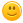21 views
I used to too.

_(\_/)
,((((^`\
((((  (6 \
,((((( ,    \
,,,_              ,(((((  /"._  ,`,
((((\\ ,...       ,((((   /    `-.-'
)))  ;'    `"'"'""((((   (
(((  /            (((      \
)) |                      |
((  |        .       '     |
))  \     _ '      `t   ,.')
(   |   y;- -,-""'"-.\   \/
)   / ./  ) /         `\  \
|./   ( (           / /'
||     \\          //'|
jgs ||      \\       _//'||
||       ))     |_/  ||
\_\     |_/          ||
`'"                  \_\
`'"

That horse is so so so so so so cool